# ISEE Middle Level Math : How to multiply variables

## Example Questions

← Previous 1 3 4 5 6 7 8

### Example Question #1 : How To Multiply Variables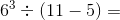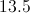Explanation:

First find the exponent value: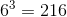Then find the value of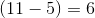Finally, solve the entire expression with the known values: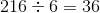### Example Question #1 : How To Multiply Variables

Simplify: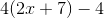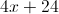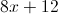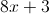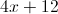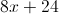Explanation: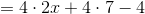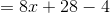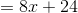### Example Question #3 : Algebraic Concepts

Multiply: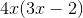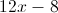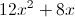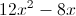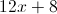Explanation:

Use the distributive property: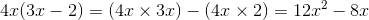### Example Question #2 : Algebraic Concepts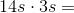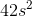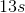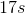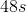Explanation:

Multiply the numbers and multiply the variables: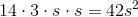Answer:### Example Question #1 : Variables

Simplify: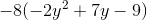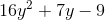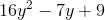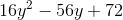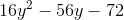Explanation: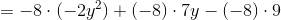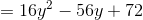### Example Question #2 : Variables

Multiply: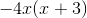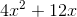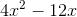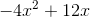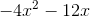Explanation: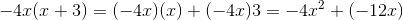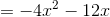### Example Question #3 : Variables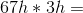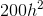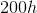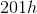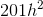Explanation:

Multiply the whole numbers and add an exponent to the variable totaling the number of exponents in the equation: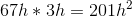Answer:### Example Question #1 : Operations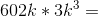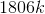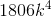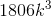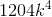Explanation:

Multiply the whole numbers and add an exponent to the variable totaling the number of exponents in the equation: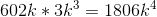Answer:### Example Question #651 : Concepts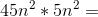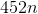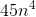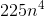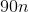Explanation:

Multiply the whole numbers and add an exponent to the variable totaling the number of exponents in the equation: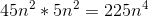Answer:### Example Question #4 : Variables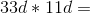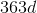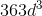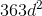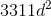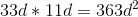Answer: